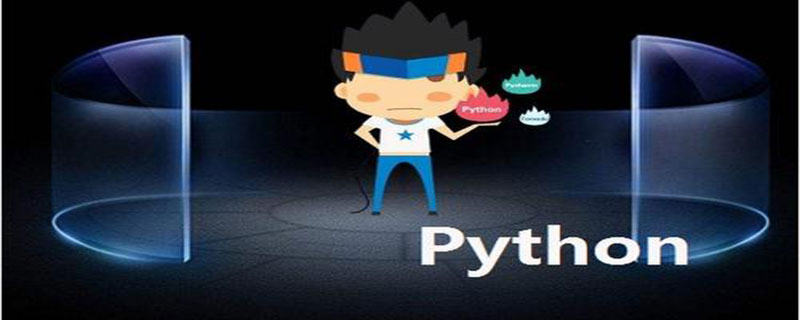## python字符串是对象吗

241次阅读python中一切都是对象

```i = 1
s = "abcde"
def foo(): pass
class C(object): pass
instance = C()
l = [1,2]
t = (1,2)```

```i = 1              #新建一个PyIntObject对象,然后绑定到i上
s = "abcde"        #新建一个PyStringObject对象，绑定到s上
def foo(): pass    #新建一个PyFunctionObject对象， 绑定到foo上
class C(object): pass    #新建一个类对象，绑定到C上
instance = C()           #新建一个实例对象，绑定到instance上
l = [1,2]                #新建一个PyListObject对象，绑定到l上
t = (1,2)                #新建一个PyTupleObject对象，绑定到t上```

```#!/usr/bin/env Python
# coding=utf-8
'''
Created on 2017年8月11日
'''
def foo(text):
return len(text)

#1.函数存入变量
f=foo

#2.函数作为参数
def show(func):
size = func("python 之禅") # 等效于 foo("Python之禅")
print ("length of string is : %s" % size)

#3.函数作为返回值
def nick():
return foo

show(f)
print(nick()("abc"))

#4.函数在执行期创造
exec('''def foo2():
print("haha")
foo2()''')

print(foo("12345"))

#5.没有被系结至某一名称的匿名函数lambda
print(map(lambda x: x * x, [1, 2, 3, 4, 5, 6, 7, 8, 9]))```